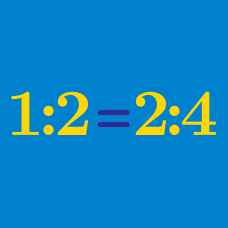Everyday Math

# Inverse Variation

The value of $y$ varies inversely with $x.$ If $y=6$ when $\displaystyle x=\frac{8}{3},$ what is the value of $y$ when $x=5 ?$

Suppose there is a water faucet that can fill a $575$-liter-tank in $100$ minutes. How many faucets do we need to fill this tank in $4$ minutes?

A cruise ship rents for $\800$ a day. If $8$ people go on a one-day trip by this ship, what is the cost per capita?

Calvin is planning to visit his hometown during Thanksgiving Holidays. It will take $9$ hours if he drives at $85 \text{ km/h}.$ How long will it take if he drives at $102 \text{ km/h} ?$

If $z$ varies directly as $x$ and inversely as $y,$ how many times larger does $z$ get if $x$ is increased by $2$ times and $y$ is decreased by $7$ times?

×